maths > construction-high

Construction of Tangents and Chord for a Circle

what you'll learn...

overview

•  Construction of a Tangent on a Point on a Circle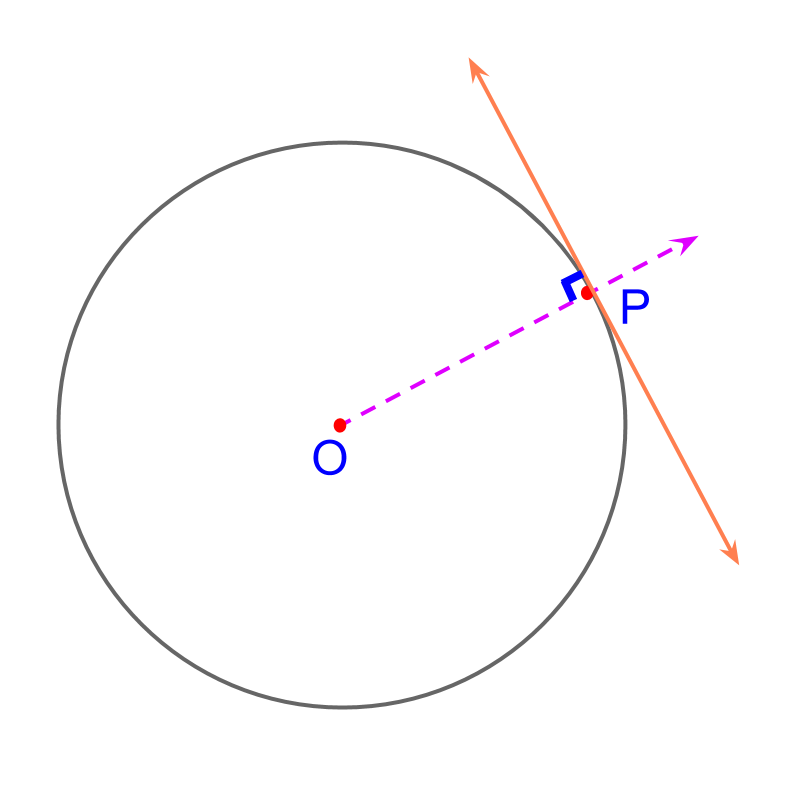•  Construction of Tangents from a Point outside a Circle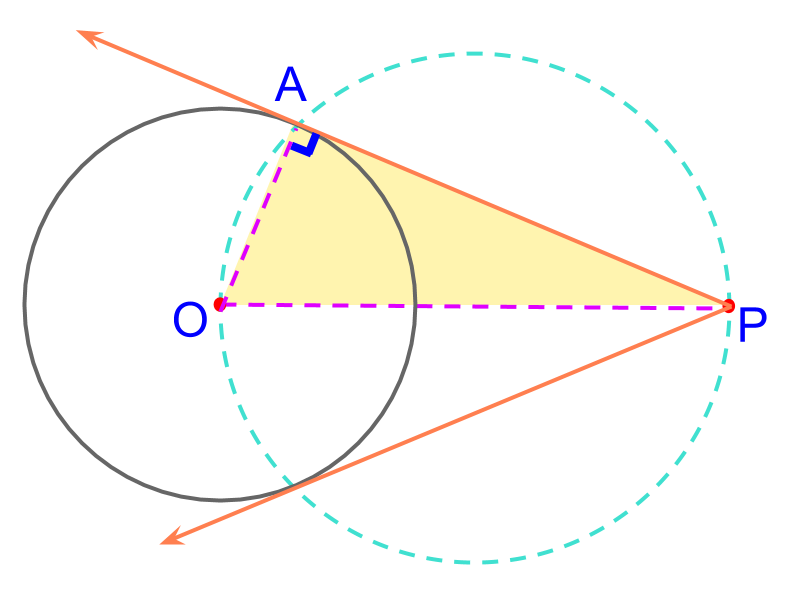•  Construction of chords of given length on a Circle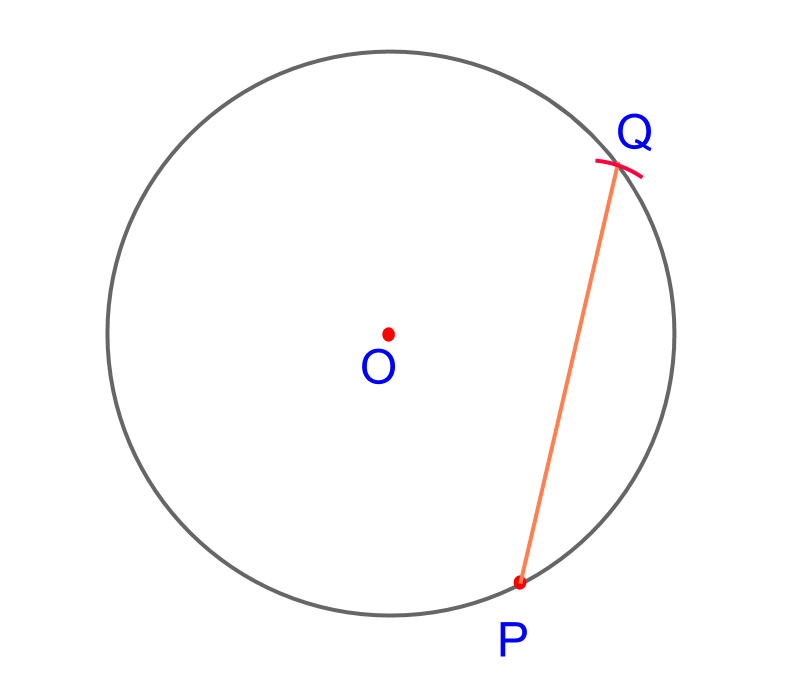•  Construction of chords at a given distance from center of a Circle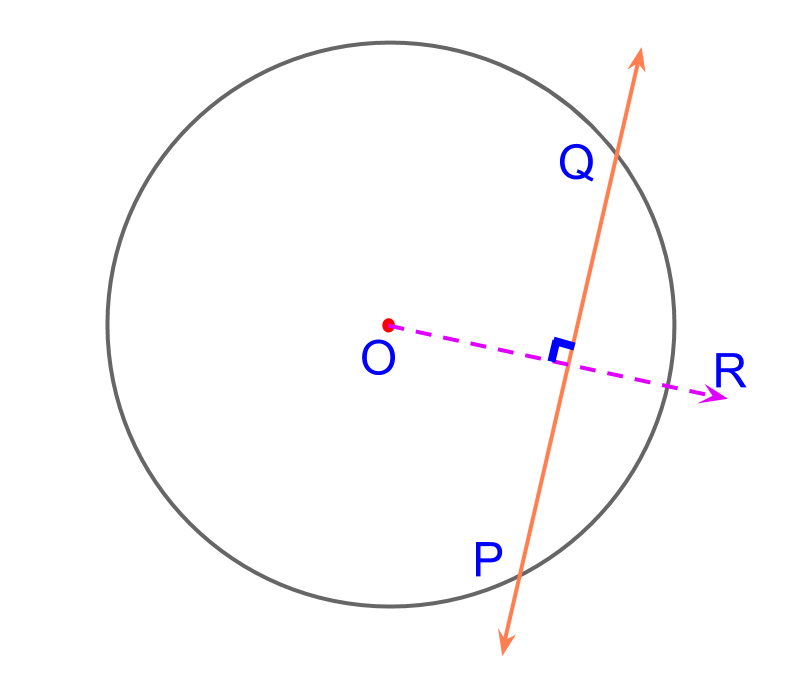tangent to a circle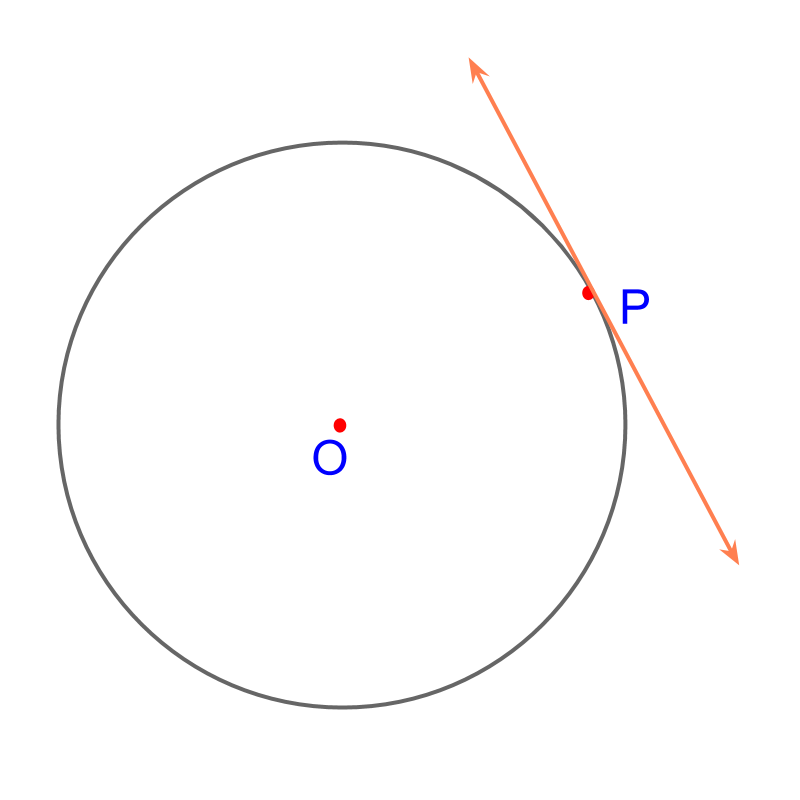Consider the circle centered at $O$$O$. The objective is to construct a tangent on the given point $P$$P$ on the circle.

To achieve that, we use the known property "tangent is at right angle ${90}^{\circ }$${90}^{\circ}$ to the radius at the point".Considering the construction of tangent on the given point $P$$P$ on a circle.

It is noted that the tangent is at right angle to the radius at the point. The ray $\stackrel{\to }{OP}$$\vec{O P}$ is constructed. Construct a perpendicular on point $P$$P$ on the ray $\stackrel{\to }{OP}$$\vec{O P}$. This construction problem is modified to the known problem : construction of a perpendicular on a point in a line.

The following steps detail construction of tangent on point $P$$P$ on a circle:

•  The ray $\stackrel{\to }{OP}$$\vec{O P}$ is constructed

•  On the point $P$$P$, the perpendicular to $\stackrel{\to }{OP}$$\vec{O P}$ is constructed.
(Construction of a perpendicular on a point is explained in the earlier lessons)

The tangent is constructed.

summaryConstruction of Tangent on a point on circle: Using the property that the radius and tangent on a point are at right angle, the construction is modified to construction of perpendicular to a line on a point.

tangent from outside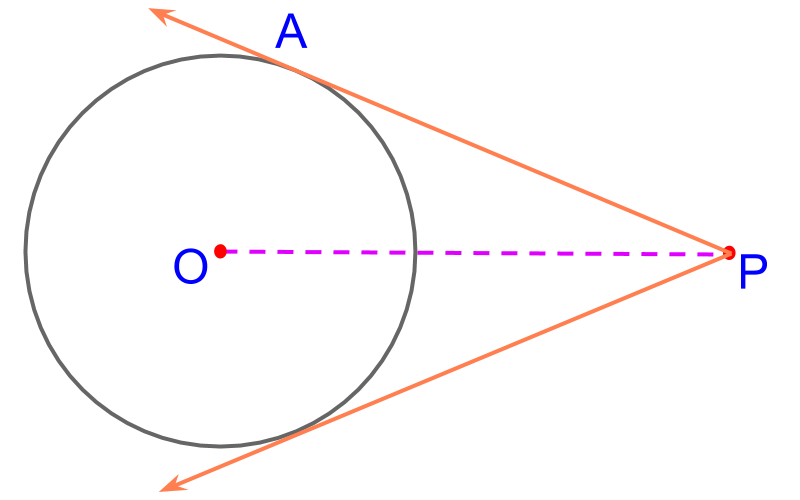Consider the circle centered at $O$$O$. The objective is to construct a tangent from the given point $P$$P$ outside the circle. To achieve that we use the property, tangent is at right angle ${90}^{\circ }$${90}^{\circ}$ to the radius at the point of tangent.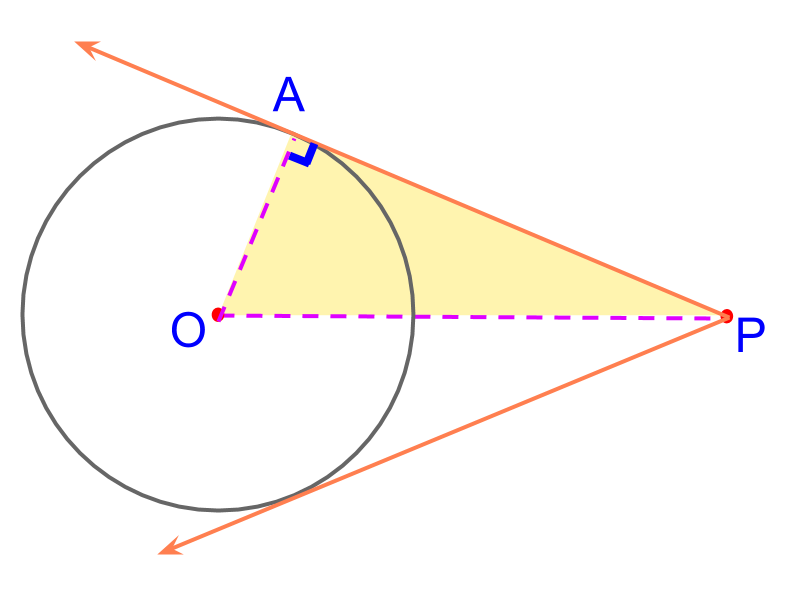Considering the construction of tangent from the given point $P$$P$ outside the circle.

The point of tangent on the circle is visualized as point $A$$A$. It is shown in the figure to illustrate. The point $A$$A$ is not yet marked. It is noted that $△OAP$$\triangle O A P$ is a right angled triangle with $\overline{OP}$$\overline{O P}$ as the hypotenuse.

To mark the point $A$$A$, "constructing a circle with hypotenuse as diameter".The point $A$$A$ is not yet marked. It is noted that $△OAP$$\triangle O A P$ is a right angled triangle with $\overline{OP}$$\overline{O P}$ as the hypotenuse.

The angle subtended by a diameter on the circle is right-angled.

Using this knowledge, It is visualized that a circle with diameter $\overline{OP}$$\overline{O P}$ intersects the given circle at point $A$$A$, where angle $\angle OAP$$\angle O A P$ is ${90}^{\circ }$${90}^{\circ}$ and so, $\stackrel{\to }{PA}$$\vec{P A}$ is the tangent at point $A$$A$.

The following steps detail construction of tangent from point $P$$P$ outside a circle:

•  The ray $\stackrel{\to }{OP}$$\vec{O P}$ is constructed

•  A circle is constructed with $\overline{OP}$$\overline{O P}$ as the diameter.
(Construction of a circle with a given line segment as diameter is explained in the earlier lessons)

•  The points of intersection of the given circle to the constructed circle are connected to $P$$P$ as the tangents of the given circle.

The tangents are constructed.

summaryConstruction of Tangents from a point outside a Circle : Note the following properties

•  the radius and tangent are perpendicular,

•  the angle subtended by diameter on a circle is right-angle

the line segment between the center of the circle and the given point is considered as diameter.

The construction is modified to construction of a circle on a given line segment as diameter.

chord of length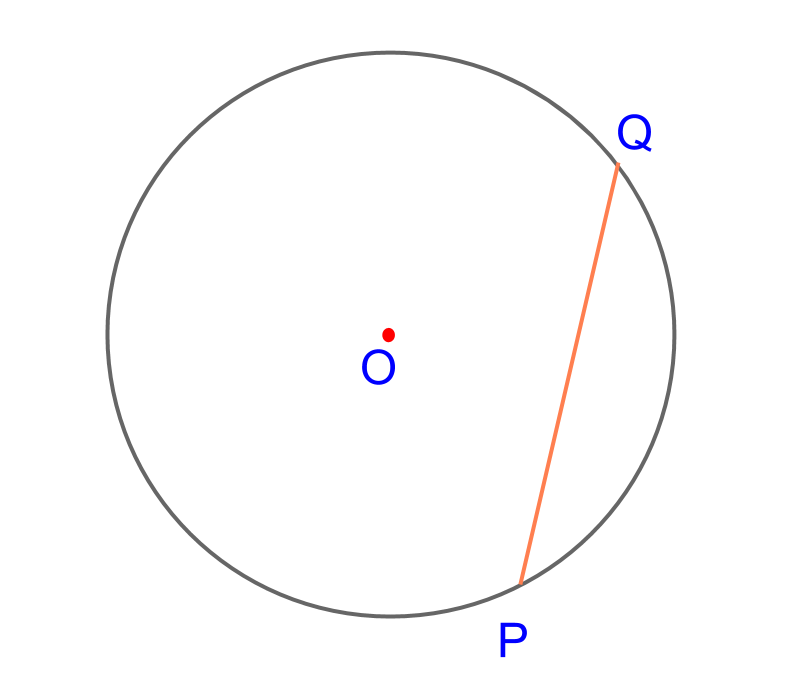Consider the circle centered at $O$$O$. The objective is to construct a chord of length $\overline{PQ}=4$$\overline{P Q} = 4$cm on the given point $P$$P$ on the circle.

To achieve that a compass is enough. A compass constructs equidistant points.The following steps detail construction of chord at point $P$$P$ on a circle:

•  Use a compass to construct an arc of length $4$$4$ cm.

•  The point of intersection of the arc on the circle is marked $Q$$Q$.

•  Connect the points $P$$P$ and $Q$$Q$

The chord $\overline{PQ}$$\overline{P Q}$ is constructed.

Note: There are two chords possible from the given point $P$$P$ of the given length.

summaryConstruction of Chords of given length : An arc of the given length is used to locate the points on circle and the chord is constructed.

chord at distance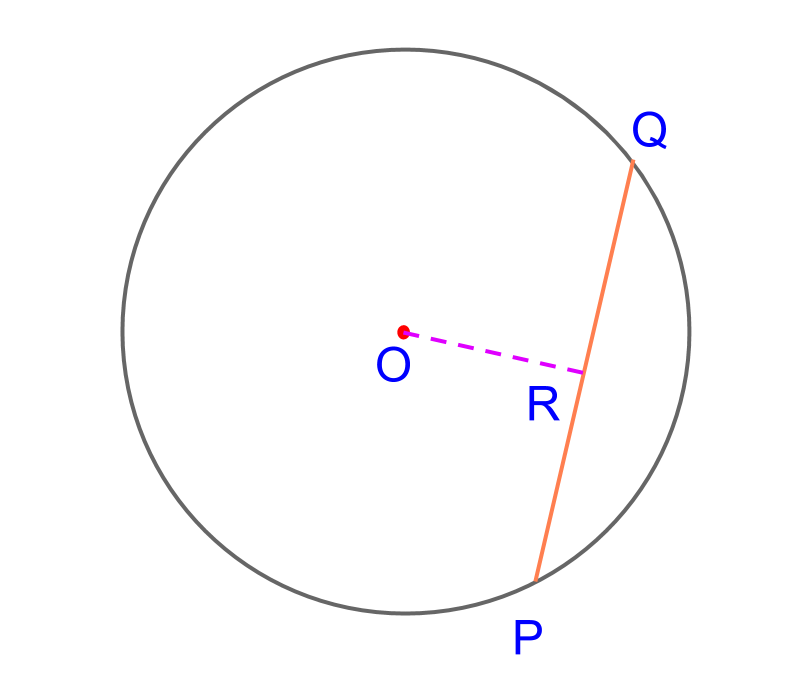Consider the circle centered at $O$$O$. The objective is to construct a chord at $2$$2$cm distance from the center. To achieve that we use the definition : the distance of chord from the center is length of the perpendicular line from the center.The following steps detail construction of chord at a distance:

•  Construct a ray $\stackrel{\to }{OR}$$\vec{O R}$.

•  Using a compass, measure the given $2$$2$cm distance and mark on the ray $\stackrel{\to }{OR}$$\vec{O R}$.

•  At the point of intersection, construct a perpendicular $\stackrel{\to }{PQ}$$\vec{P Q}$

•  The ray intersects at points $P$$P$ and $Q$$Q$

The chord $\overline{PQ}$$\overline{P Q}$ is constructed.

summaryConstruction of Chord at a distance from Center of the circle : Using the property that the distance of a chord from the center is measured as the perpendicular distance, a perpendicular is constructed on a radius at the given distance.

Outline

The outline of material to learn "Consrtruction (High school)" is as follows.